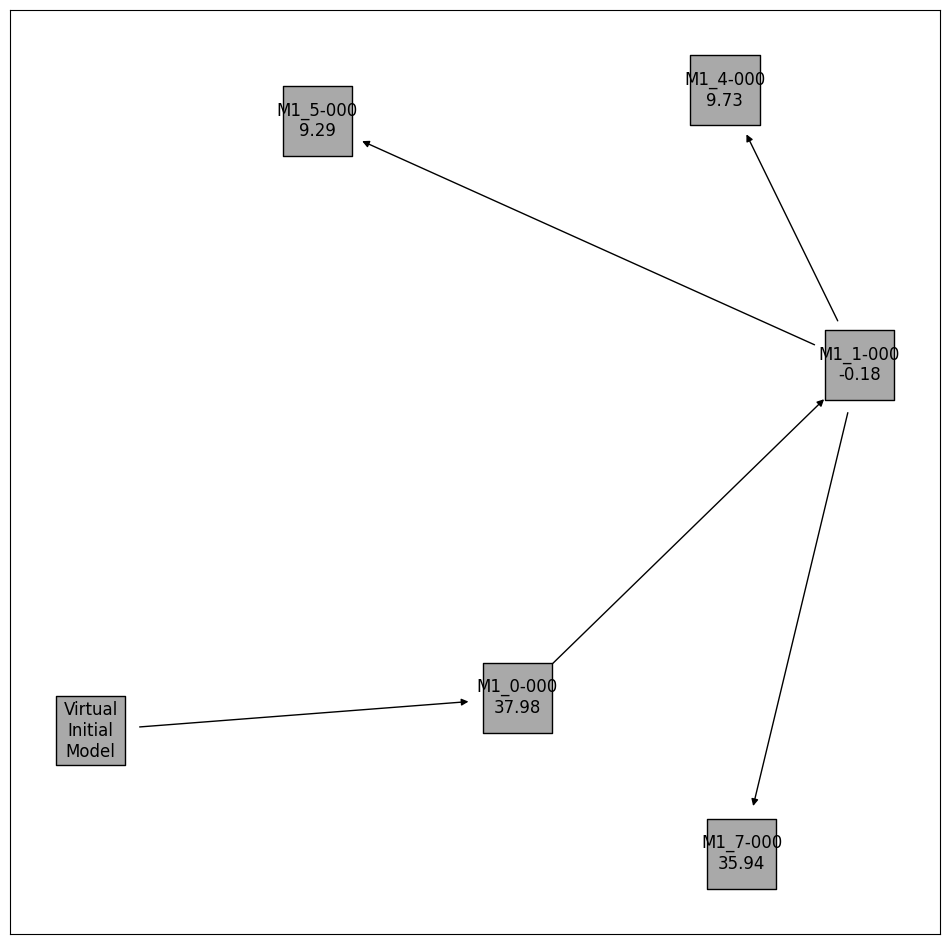# Model Selection

In this notebook, the model selection capabilities of pyPESTO are demonstrated, which facilitate the selection of the best model from a set of possible models. This includes examples of forward, backward, and brute force methods, as well as criteria such as AIC, AICc, and BIC. Various additional options and convenience methods are also demonstrated.

All specification files use the PEtab Select format, which is a model selection extension to the parameter estimation specification format PEtab.

Dependencies can be installed with pip3 install pypesto[select].

In this notebook:

## Example Model

This example involves a reaction system with two species (A and B), with their growth, conversion and decay rates controlled by three parameters ($$\theta_1$$, $$\theta_2$$, and $$\theta_3$$). Many different hypotheses will be considered, which are described in the model specifications file. There, a parameter fixed to zero indicates that the associated reaction(s) should not be in the model which resembles a hypothesis.

Synthetic measurement data is used here, which was generated with the “true” model. The comprehensive model includes additional behavior involving a third parameter ($$\theta_3$$). Hence, during model selection, models without $$\theta_3=0$$ should be preferred.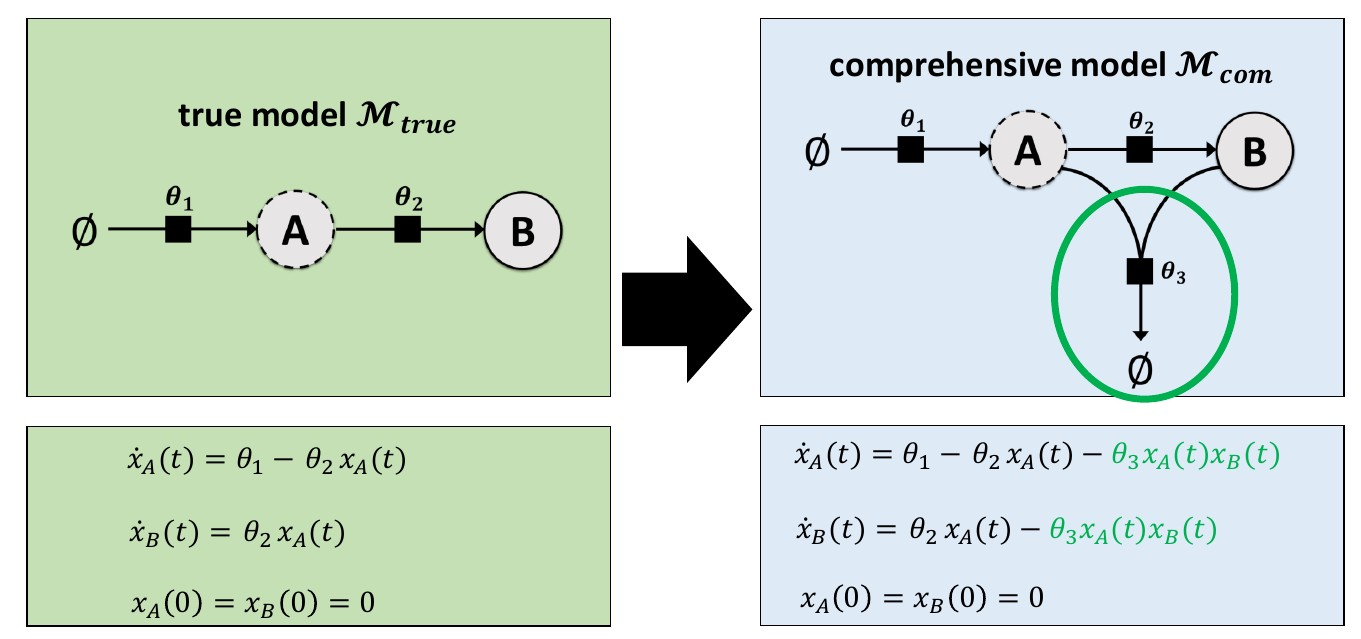## Model Space Specifications File

The model selection specification file can be written in the following compressed format.

model_subspace_id

petab_yaml

$$\theta_1$$

$$\theta_2$$

$$\theta_3$$

M1

example_modelSelection.yaml

0;estimate

0;estimate

0;estimate

Alternatively, models can be explicitly specified. The below table is equivalent to the above table.

model_subspace_id

petab_yaml

$$\theta_1$$

$$\theta_2$$

$$\theta_3$$

M1_0

example_modelSelection.yaml

0

0

0

M1_1

example_modelSelection.yaml

0

0

estimate

M1_2

example_modelSelection.yaml

0

estimate

0

M1_3

example_modelSelection.yaml

estimate

0

0

M1_4

example_modelSelection.yaml

0

estimate

estimate

M1_5

example_modelSelection.yaml

estimate

0

estimate

M1_6

example_modelSelection.yaml

estimate

estimate

0

M1_7

example_modelSelection.yaml

estimate

estimate

estimate

Either of the above tables (as TSV files) are valid inputs. Any combinations of cells in the compressed or explicit format is also acceptable, including the following example.

model_subspace_id

petab_yaml

$$\theta_1$$

$$\theta_2$$

$$\theta_3$$

M1

example_modelSelection.yaml

0;estimate

0;estimate

0

M2

example_modelSelection.yaml

0;estimate

0;estimate

estimate

Due to the topology of the example model, setting $$\theta_1$$ to zero can result in a model with no dynamics. Hence, for this example, some parameters are set to non-zero fixed values. These parameters are considered as fixed (not estimated) values in criterion (e.g. AIC) calculations.

The model specification table used in this notebook is shown below.

:

import pandas as pd
from IPython.display import HTML, display

display(HTML(df.to_html(index=False)))

model_subspace_id petab_yaml k1 k2 k3
M1_0 example_modelSelection.yaml 0 0 0
M1_1 example_modelSelection.yaml 0.2 0.1 estimate
M1_2 example_modelSelection.yaml 0.2 estimate 0
M1_3 example_modelSelection.yaml estimate 0.1 0
M1_4 example_modelSelection.yaml 0.2 estimate estimate
M1_5 example_modelSelection.yaml estimate 0.1 estimate
M1_6 example_modelSelection.yaml estimate estimate 0
M1_7 example_modelSelection.yaml estimate estimate estimate

## Forward Selection, Multiple Searches

Here, we show a typical workflow for model selection. First, a PEtab Select problem is created, which is used to initialize a pyPESTO model selection problem.

:

from petab_select import VIRTUAL_INITIAL_MODEL

# Helpers for plotting etc.
def get_labels(models):
labels = {
model.get_hash(): str(model.model_subspace_id)
for model in models
if model != VIRTUAL_INITIAL_MODEL
}
return labels

def get_digraph_labels(models, criterion):
zero = min(model.get_criterion(criterion) for model in models)
labels = {
model.get_hash(): f"{model.model_subspace_id}\n{model.get_criterion(criterion) - zero:.2f}"
for model in models
}
return labels

# Disable some logged messages that make the model selection log more
import tqdm

def nop(it, *a, **k):
return it

tqdm.tqdm = nop

:

import petab_select
from petab_select import ESTIMATE, Criterion, Method

import pypesto.select

petab_select_problem = petab_select.Problem.from_yaml(
"model_selection/petab_select_problem.yaml"
)

:

import logging

import pypesto.logging

pypesto.logging.log(level=logging.WARNING, name="pypesto.petab", console=True)

pypesto_select_problem_1 = pypesto.select.Problem(
petab_select_problem=petab_select_problem
)


Models can be selected with a model selection algorithm (here: forward) and a comparison criterion (here: AIC). The forward method starts with the smallest model. Within each following iteration it tests all models with one additional estimated parameter.

To perform a single iteration, use select as shown below. Later in the notebook, select_to_completion is demonstrated, which performs multiple consecutive iterations automatically.

As no initial model is specified here, a virtual initial model with no estimated parameters is automatically used to find the “smallest” (in terms of number of estimated parameters) models. In this example, this is the model M1_0, which has no estimated parameters.

:

# Reduce notebook runtime
minimize_options = {
"n_starts": 10,
"filename": None,
"progress_bar": False,
}

best_model_1, _ = pypesto_select_problem_1.select(
method=Method.FORWARD,
criterion=Criterion.AIC,
minimize_options=minimize_options,
)

--------------------New Selection--------------------
model0       | model        | crit         | model0_crit  | model_crit   | crit_diff    | accept
virtual_init | M1_0:649bcd9 | AIC          | None         | 3.698e+01    | None         | True


To search more of the model space, hence models with more parameters, the algorithm can be repeated. As models with no estimated parameters have already been tested, subsequent select calls will begin with the next simplest model (in this case, models with exactly 1 estimated parameter, if they exist in the model space), and move on to more complex models.

The best model from the first iteration is supplied as the predecessor (initial) model here.

:

best_model_2, _ = pypesto_select_problem_1.select(
method=Method.FORWARD,
criterion=Criterion.AIC,
minimize_options=minimize_options,
predecessor_model=best_model_1,
)

--------------------New Selection--------------------
model0       | model        | crit         | model0_crit  | model_crit   | crit_diff    | accept
M1_0:649bcd9 | M1_1:9202ace | AIC          | 3.698e+01    | -4.175e+00   | -4.115e+01   | True
M1_0:649bcd9 | M1_2:c773362 | AIC          | 3.698e+01    | -4.275e+00   | -4.125e+01   | True
M1_0:649bcd9 | M1_3:e03f5dc | AIC          | 3.698e+01    | -4.705e+00   | -4.168e+01   | True


Plotting routines to visualize the best model at each iteration of the selection process, or to visualize the graph of models that have been visited in the model space are available.

:

import pypesto.visualize.select as pvs

selected_models = [best_model_1, best_model_2]
ax = pvs.plot_selected_models(
[best_model_1, best_model_2],
criterion=Criterion.AIC,
relative=False,
labels=get_labels(selected_models),
)
ax = pvs.plot_selected_models(
[best_model_1, best_model_2],
criterion=Criterion.AIC,
labels=get_labels(selected_models),
)
ax.plot();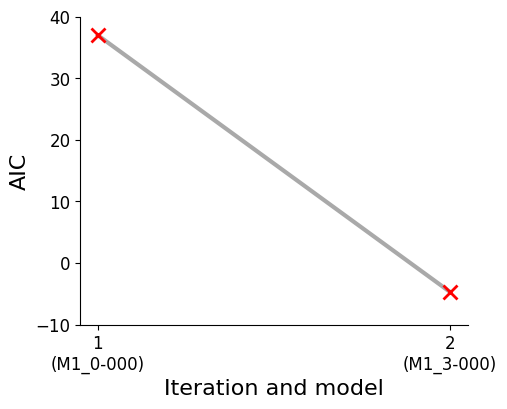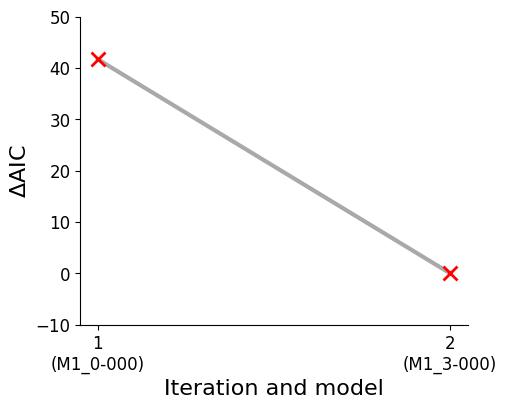:

pvs.plot_calibrated_models_digraph(
problem=pypesto_select_problem_1,
labels=get_digraph_labels(
pypesto_select_problem_1.calibrated_models.values(),
criterion=Criterion.AIC,
),
);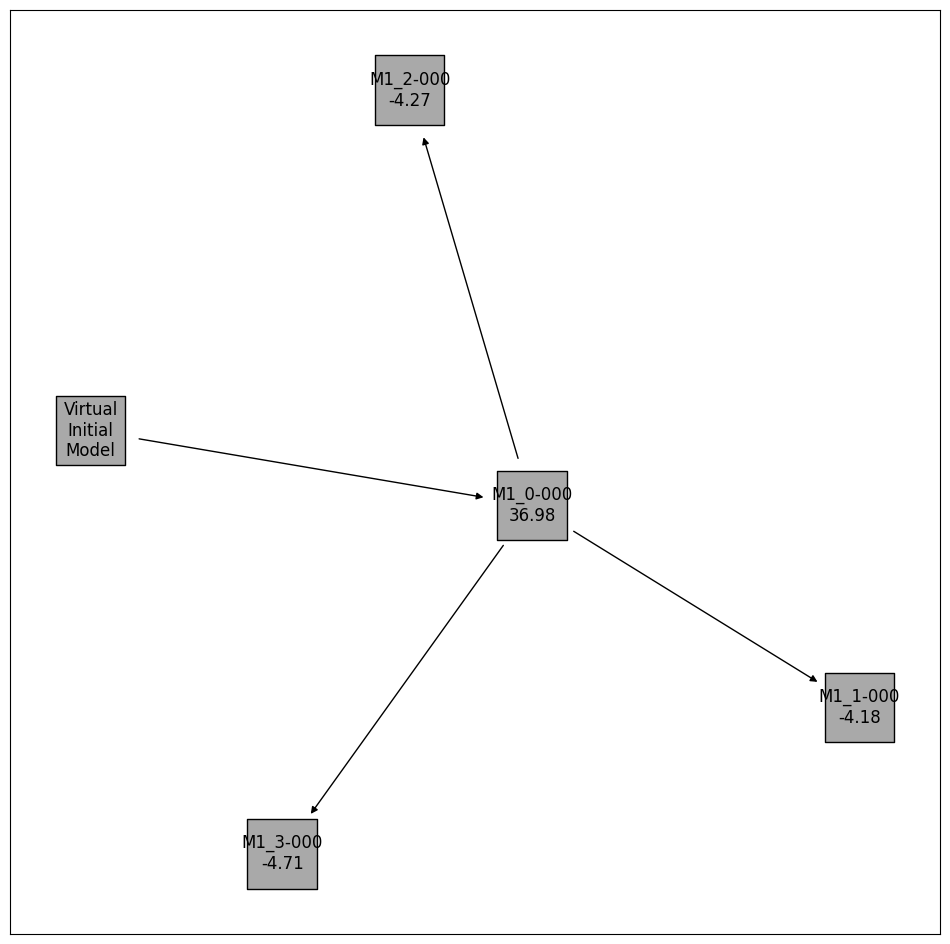## Backward Selection, Custom Initial Model

Backward selection is specified by changing the algorithm from Method.FORWARD to Method.BACKWARD in the select() call.

A custom initial model is specified with the optional predecessor_model argument of select().

:

import numpy as np
from petab_select import Model

petab_select_problem.model_space.reset_exclusions()
pypesto_select_problem_2 = pypesto.select.Problem(
petab_select_problem=petab_select_problem
)

petab_yaml = "model_selection/example_modelSelection.yaml"
initial_model = Model(
model_id="myModel",
petab_yaml=petab_yaml,
parameters=dict(
k1=0.1,
k2=ESTIMATE,
k3=ESTIMATE,
),
criteria={petab_select_problem.criterion: np.inf},
)

print("Initial model:")
print(initial_model)

Initial model:
model_id        petab_yaml      k1      k2      k3
myModel model_selection/example_modelSelection.yaml     0.1     estimate        estimate

:

pypesto_select_problem_2.select(
method=Method.BACKWARD,
criterion=Criterion.AIC,
predecessor_model=initial_model,
minimize_options=minimize_options,
);

--------------------New Selection--------------------
model0       | model        | crit         | model0_crit  | model_crit   | crit_diff    | accept
:myModel     | M1_1:9202ace | AIC          | inf          | 3.897e+01    | -inf         | True
:myModel     | M1_2:c773362 | AIC          | inf          | 2.410e+01    | -inf         | True

:

initial_model_label = {initial_model.get_hash(): initial_model.model_id}

pvs.plot_calibrated_models_digraph(
problem=pypesto_select_problem_2,
labels={
**get_digraph_labels(
pypesto_select_problem_2.calibrated_models.values(),
criterion=Criterion.AIC,
),
**initial_model_label,
},
);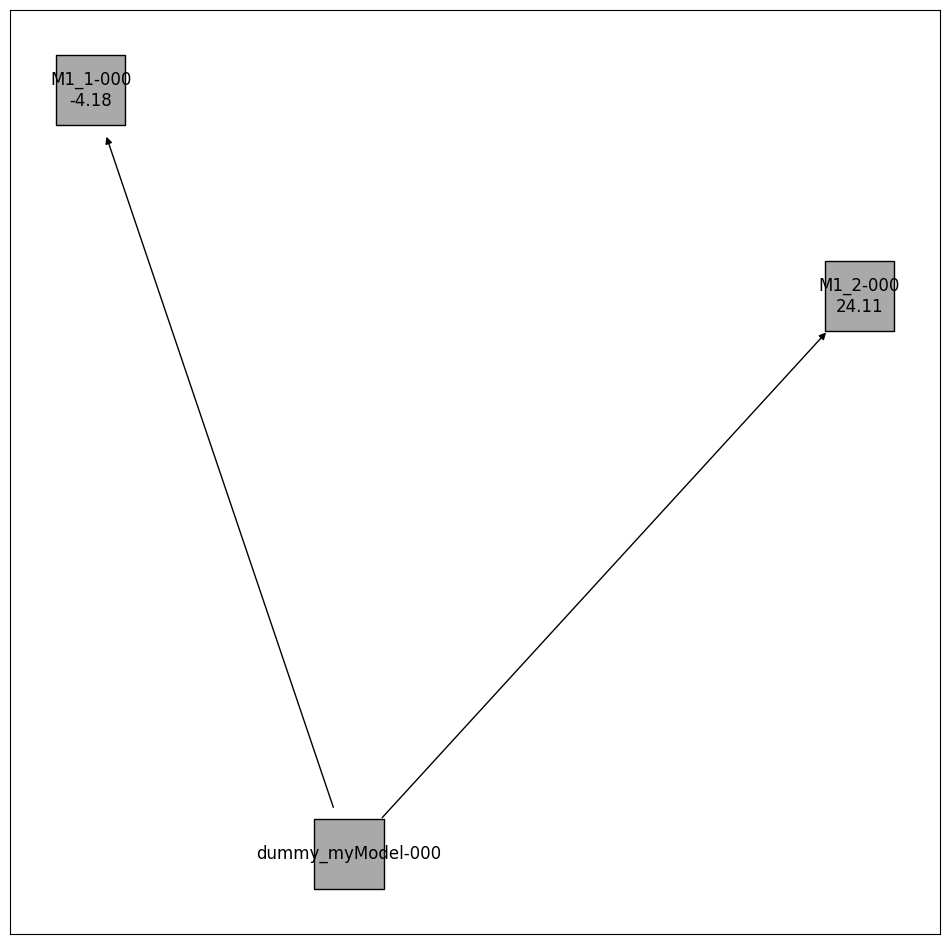There exist additional options that can be used to further customise selection algorithms.

### Select First Improvement

At each selection step, as soon as a model that improves on the previous model is encountered (by the specified criterion), it is selected and immediately used as the previous model in the next iteration of the selection. This is unlike the default behaviour, where all test models at each iteration are optimized, and the best of these is selected.

### Use Previous Maximum Likelihood Estimate as Startpoint

The maximum likelihood estimate from the previous model is used as one of the startpoints in the multistart optimization of the test models. The default behaviour is that all startpoints are automatically generated by pyPESTO.

### Minimize Options

Optimization can be customised with a dictionary that specifies values for the corresponding keyword arguments of minimize.

### Criterion Options

Currently implemented options are: Criterion.AIC (Akaike information criterion), Criterion.AICC (corrected AIC), and Criterion.BIC (Bayesian information criterion).

#### Criterion Threshold

A threshold can be specified, such that only models that improve on previous models by the threshold amount in the chosen criterion are accepted.

:

petab_select_problem.model_space.reset_exclusions()
pypesto_select_problem_3 = pypesto.select.Problem(
petab_select_problem=petab_select_problem
)
best_models = pypesto_select_problem_3.select_to_completion(
method=Method.FORWARD,
criterion=Criterion.BIC,
select_first_improvement=True,
startpoint_latest_mle=True,
minimize_options=minimize_options,
)

--------------------New Selection--------------------
model0       | model        | crit         | model0_crit  | model_crit   | crit_diff    | accept
virtual_init | M1_0:649bcd9 | BIC          | None         | 3.677e+01    | None         | True
--------------------New Selection--------------------
model0       | model        | crit         | model0_crit  | model_crit   | crit_diff    | accept
M1_0:649bcd9 | M1_1:9202ace | BIC          | 3.677e+01    | -4.592e+00   | -4.136e+01   | True
--------------------New Selection--------------------
model0       | model        | crit         | model0_crit  | model_crit   | crit_diff    | accept
M1_1:9202ace | M1_4:3d668e2 | BIC          | -4.592e+00   | -2.899e+00   | 1.693e+00    | False
M1_1:9202ace | M1_5:d2503eb | BIC          | -4.592e+00   | -3.330e+00   | 1.262e+00    | False
--------------------New Selection--------------------
model0       | model        | crit         | model0_crit  | model_crit   | crit_diff    | accept
M1_1:9202ace | M1_7:afa580e | BIC          | -4.592e+00   | -4.889e+00   | -2.973e-01   | True
--------------------New Selection--------------------
model0       | model        | crit         | model0_crit  | model_crit   | crit_diff    | accept

:

pvs.plot_selected_models(
selected_models=best_models,
criterion=Criterion.BIC,
labels=get_labels(best_models),
);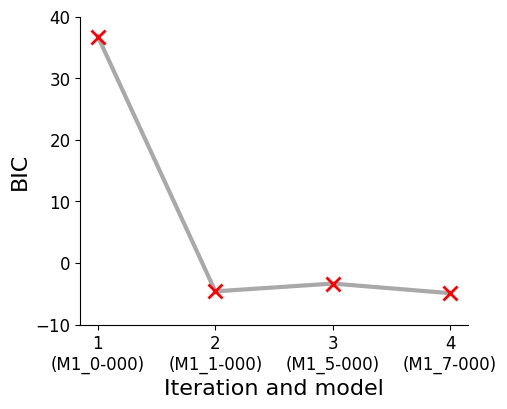:

pvs.plot_calibrated_models_digraph(
problem=pypesto_select_problem_3,
criterion=Criterion.BIC,
relative=False,
labels=get_digraph_labels(
pypesto_select_problem_3.calibrated_models.values(),
criterion=Criterion.BIC,
),
);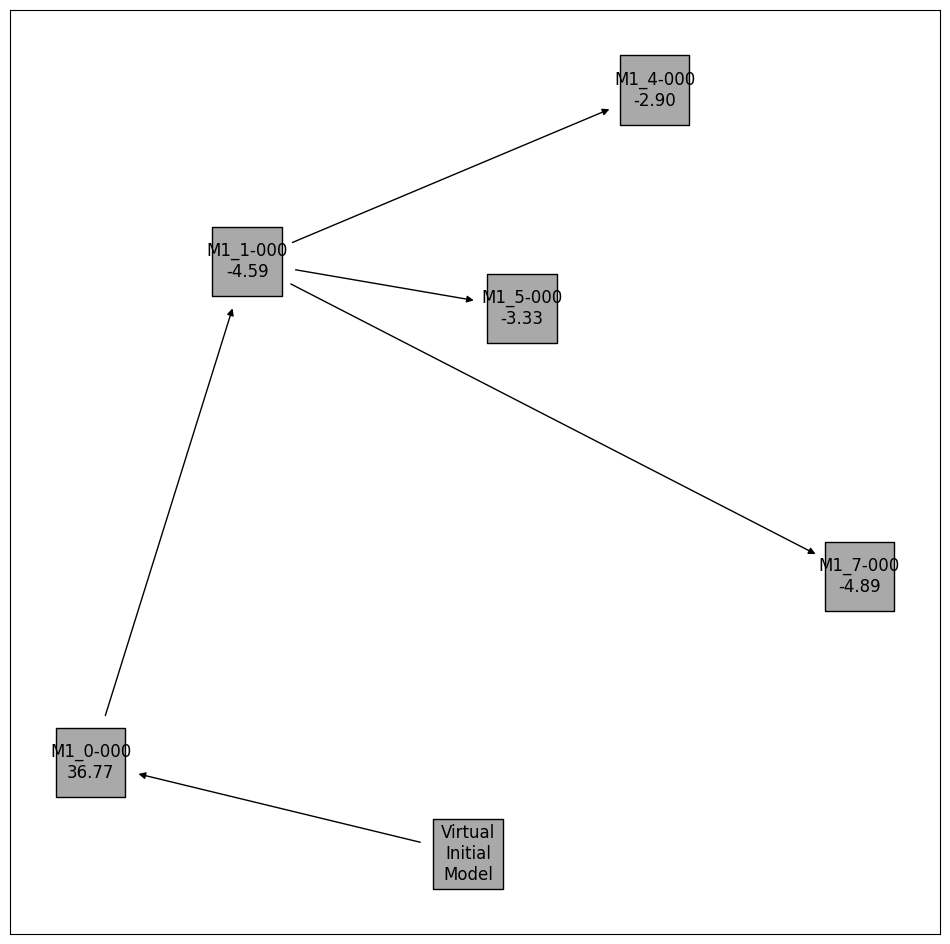:

# Repeat with AICc and criterion_threshold == 10
petab_select_problem.model_space.reset_exclusions()
pypesto_select_problem_4 = pypesto.select.Problem(
petab_select_problem=petab_select_problem
)
best_models = pypesto_select_problem_4.select_to_completion(
method=Method.FORWARD,
criterion=Criterion.AICC,
select_first_improvement=True,
startpoint_latest_mle=True,
minimize_options=minimize_options,
criterion_threshold=10,
)

--------------------New Selection--------------------
model0       | model        | crit         | model0_crit  | model_crit   | crit_diff    | accept
virtual_init | M1_0:649bcd9 | AICc         | None         | 3.798e+01    | None         | True
--------------------New Selection--------------------
model0       | model        | crit         | model0_crit  | model_crit   | crit_diff    | accept
M1_0:649bcd9 | M1_1:9202ace | AICc         | 3.798e+01    | -1.754e-01   | -3.815e+01   | True
--------------------New Selection--------------------
model0       | model        | crit         | model0_crit  | model_crit   | crit_diff    | accept
M1_1:9202ace | M1_4:3d668e2 | AICc         | -1.754e-01   | 9.725e+00    | 9.901e+00    | False
M1_1:9202ace | M1_5:d2503eb | AICc         | -1.754e-01   | 9.295e+00    | 9.470e+00    | False
--------------------New Selection--------------------
model0       | model        | crit         | model0_crit  | model_crit   | crit_diff    | accept
M1_1:9202ace | M1_7:afa580e | AICc         | -1.754e-01   | 3.595e+01    | 3.612e+01    | False
--------------------New Selection--------------------
model0       | model        | crit         | model0_crit  | model_crit   | crit_diff    | accept

:

pvs.plot_selected_models(
selected_models=best_models,
criterion=Criterion.AICC,
labels=get_labels(best_models),
);:

pvs.plot_calibrated_models_digraph(
problem=pypesto_select_problem_4,
criterion=Criterion.AICC,
relative=False,
labels=get_digraph_labels(
pypesto_select_problem_4.calibrated_models.values(),
criterion=Criterion.AICC,
),
);## Multistart

Multiple model selections can be run by specifying multiple initial models.

:

petab_select_problem.model_space.reset_exclusions()
pypesto_select_problem_5 = pypesto.select.Problem(
petab_select_problem=petab_select_problem
)

initial_model_1 = Model(
model_id="myModel1",
petab_yaml=petab_yaml,
parameters=dict(
k1=0,
k2=0,
k3=0,
),
criteria={petab_select_problem.criterion: np.inf},
)

initial_model_2 = Model(
model_id="myModel2",
petab_yaml=petab_yaml,
parameters=dict(
k1=ESTIMATE,
k2=ESTIMATE,
k3=0,
),
criteria={petab_select_problem.criterion: np.inf},
)

initial_models = [initial_model_1, initial_model_2]
best_model, best_models = pypesto_select_problem_5.multistart_select(
method=Method.FORWARD,
criterion=Criterion.AIC,
predecessor_models=initial_models,
minimize_options=minimize_options,
)

--------------------New Selection--------------------
model0       | model        | crit         | model0_crit  | model_crit   | crit_diff    | accept
:myModel1    | M1_1:9202ace | AIC          | inf          | 3.897e+01    | -inf         | True
:myModel1    | M1_2:c773362 | AIC          | inf          | -4.275e+00   | -inf         | True
:myModel1    | M1_3:e03f5dc | AIC          | inf          | -4.705e+00   | -inf         | True
--------------------New Selection--------------------
model0       | model        | crit         | model0_crit  | model_crit   | crit_diff    | accept
:myModel2    | M1_7:afa580e | AIC          | inf          | 4.011e+01    | -inf         | True

:

initial_model_labels = {
initial_model.get_hash(): initial_model.model_id
for initial_model in initial_models
}

pvs.plot_calibrated_models_digraph(
problem=pypesto_select_problem_5,
criterion=Criterion.AIC,
relative=False,
labels={
**get_digraph_labels(
pypesto_select_problem_5.calibrated_models.values(),
criterion=Criterion.AIC,
),
**initial_model_labels,
},
);